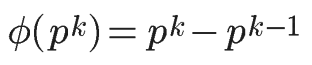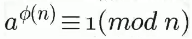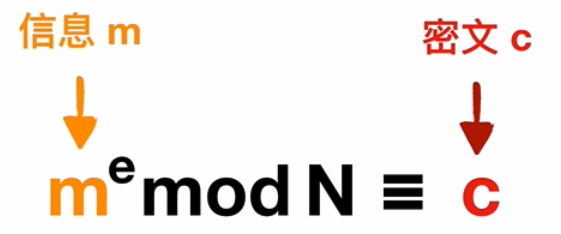# 简介• RSA是目前最有影响力和最常用的公钥加密算法，它能够抵抗到目前为止已知的绝大多数密码攻击，已被ISO推荐为公钥数据加密标准。

• RSA算法基于一个十分简单的数论事实：将两个大质数相乘十分容易，但是想要对其乘积进行因式分解却极其困难，因此可以将乘积公开。

• 本文将介绍RSA算法所需的知识基础、RSA算法基本原理。

# 知识储备

• RSA算法加解密其实就是两个公式，但是为了理解这两个公式我们需要学习数论中的四个概念：`互质、欧拉函数、欧拉定理、模反元素`，这四个概念都是非常好理解的，只需要有初高中的数学知识就能理解。

## 互质关系

• 互质整数是公约数只有1的两个整数,比如15和32,这说明不是质数也可以构成互质关系。

• 关于互质关系,有以下结论:

1. 任意两个质数构成互质关系，比如13和61
2. 一个数是质数，另一个数只要不是前者的倍数，两者就构成互质关系，比如3和10
3. 如果两个数之中，较大的那个数是质数，则两者构成互质关系，比如97和57
4. p是大于1的奇数，则p和p-2构成互质关系，比如27和25
5. p是大于1的整数，则p和p-1构成互质关系，比如53和52
6. 1和任意一个自然数是都是互质关系，比如1和99

## 欧拉函数

• 欧拉函数听起来很高大上，但其实非常简单，欧拉函数是小于x的整数中与x互质的数的个数，一般用φ(x)表示。例如φ(8)就等于4,因为8以内的与8互质的数只有1、3、5、7这四个数。

• 关于欧拉函数有几点性质:

1. 特殊的，`φ(1)=1`
2. 如果n是质数，则 `φ(n)=n-1` 。因为质数与小于它的每一个数，都构成互质关系。比如5与1、2、3、4都构成互质关系。
3. 如果n是质数的某一个次方，即 n = p^k (p为质数，k为大于等于1的整数)，则比如 φ(8) = φ(2^3) =2^3 – 2^2 = 8 -4 = 4。
4. 如果n可以分解成两个互质的整数之积,即`n = p1 × p2`,则`φ(n) = φ(p1p2) = φ(p1) × φ(p2)`

## 欧拉定理

• 欧拉函数的用处，在于欧拉定理，而欧拉定理是RSA算法的核心。

• `欧拉定理`指的是: 如果两个正整数a和n互质，则n的欧拉函数 φ(n) 可以让下面的等式成立：• 其中’≡’是恒等号(在这也可叫为同余号),表示该等式始终成立而与n、a的值无关。

• 这个式子的含义为:a的φ(n)次方除以n的余数为1。

• 欧拉定理的证明较为复杂,我们只需要记住这条结论就行,挺好记的。

## 模反元素

• 如果两个正整数a和n互质，那么一定可以找到整数b(不一定唯一)，使得 ab-1 被n整除，或者说ab除以n的余数是1。

• `ab≡1 (mod n)`, 这时，b就叫做a的“乘法逆元（乘法模反元素）”。

• 比如，5和17互质，那么7就是5的模反元素,同时24、41、58…都是5的模反元素，即如果b是a的模反元素，则 `b+kn` 都是`a`的模反元素。

## 明文and密文

• 最后我们介绍一组概念。
1. 明文(Plain Text):明文，是指没有加密的文字（或者字符串），属于密码学术语。
2. 密文(Cipher Text):密文是加了密的的文字。
• 即明文是加密之前的文字。密文是对明文进行加密后的报文。

# RSA算法基本原理

• RSA是一种非对称加密算法。非对称加密算法的优点我们会在后面的RSA加解密演示中介绍到。

• 在进行RSA加密与解密之前,我们先要有RSA密钥,即公钥和私钥。

• 我们用公钥对明文进行加密, 用私钥对密文进行解密。公钥是可以让所有人都知道的,但是私钥只能我们自己知道。

## 密钥生成规则

### (1)随机选择两个不相等的质数p和q

• 我们选择61和53,在实际应用中,这两个质数越大就越难破解。

### (2)计算p和q的乘积n

• `n = 61 * 53 = 3233`
• 3233写成二进制是110010100001共12位,故该密钥是12位的,目前主流可选值：1024、2048、3072、4096…低于1024bit的密钥已经不建议使用（安全问题）。

### (3)计算n的欧拉函数φ(n)

• 因为n = p*q,由欧拉函数的小性质 ,`如果n = p × q且p、q互质,则φ(n) = φ(pq) = φ(p)φ(q)`

• 由于我们的p和q都是质数,再一次由欧拉函数的小性质`如果n是质数，则 φ(n)=n-1`,可得`φ(p) = p-1` , `φ(q) = q - 1`。所以我们的`φ(n) = (p-1)(q-1)`

• 所以我们例子中的`φ(n) = 60×52 = 3120`

### (4)按照条件随机选择一个整数e

• 选择e的条件是 `1< e < φ(n)，且e与φ(n) 互质`

• 本例中选取e = 17,但实际应用中e常常取65537 。

### (5)计算e对于φ(n)的模反元素d

• 根据欧拉定理我们有:`ed ≡ 1 ( mod φ(n) )`

• `ed–kφ(n)=1`

• `e = 17`,`φ(n) = 3120`代入可得:`17d–3120k=1`

• 这里我们采用拓展欧几里得算法求解d的值。

• 此处给出求解代码:
• 这样求出我们的`d = 2753`

### (6) 将n和e封装成公钥,n和d封装成私钥

• 前面我们已经求出来了:
1. p = 61
2. q = 53; 或者写成p = 53,q = 61都可以。
3. n = pq = 3233
4. φ(n) = (p-1)(q-1) = 3120
5. e = 17; 满足1< e < φ(n)，且e与φ(n) 互质。
6. d = 2753;e对于φ(n)的模反元素d。
• 所以我们的公钥就是`(n,e) = (3233,17)`,私钥就是`(n,d) = (3233,2753)`

### RSA算法可靠性分析

• 我们一共生成了六个数字：p、q、n、φ(n)、e、d，这六个数字之中，公钥用到了两个（n和e），其余四个数字都是不公开的。其中最关键的是d，因为n和d组成了私钥，一旦d泄漏，就等于私钥泄漏。

• 那么，有无可能在已知n和e的情况下，推导出d？

1. `ed≡1 (mod φ(n))`。只有知道e和φ(n)，才能算出d。
2. `φ(n)=(p-1)(q-1)`。只有知道p和q，才能算出φ(n)。
3. `n=pq`。只有将n因数分解，才能算出p和q。
• 综上,如果n可以被因数分解,那么就可以算出d,从而拿到私钥。

• 大数的因数分解是非常困难的。限制人类分解大整数的是计算机的计算能力，相信如果有一天真正的量子计算机问世后，又会引发一轮安全加密竞赛！

## RSA加解密演示

### 情景导入

• 我们以经典的Alice和Bob的故事来导入情景。
• Alice在学习了RSA算法以后,制作了公钥和私钥(假定和我们上面制作的公钥私钥一样),Alice将公钥发送给Bob并告诉Bob以后给自己发消息之前先用公钥进行加密，Alice自己保留私钥。

### 加密

• 假设某天Bob要给Alice发送一个字母’A’,Bob需要先将’A’转为ascii码65，所以m = 65。ps: m必须是整数(字符串可以取ascii值或unicode值)，且`m必须小于n`

• 那么我们根据下图关系可得到密文c。• 由于我们的公钥是`(n,e) = (3233,17)`,那么有`65^17≡c(mod 3233)`(本文中的’^’均表示几次方而不表示异或),这样我们求得`c = 2790`,那么Bob就可以把`c = 2790`发送给Alice了。

• 这样即使有第三者截获了密文c,或者截获了公钥和密文c,由于第三者没有私钥,很难将密文c解密到明文m。此时非对称加密的优点便体现出来了。如果看得有点迷,没关系,咱们打个比方。

• 非对称加密就像Alice将一把已经打开的锁发送给了Bob而自己留着开锁钥匙,让Bob每次给自己发送消息的时候都用这把锁将消息锁住,当Alice收到消息后用只有自己才有的钥匙来解锁得到真正的信息。这样就算第三者劫持了密文和锁,因为没有钥匙,也很难很难拿到真正的消息。

• 假设我们用的对称加密,往往会把密钥和密文一同传给接收者,就像把钥匙和密文一起发送出去,这样如果一旦有第三者截获了密文和钥匙,就很容易利用密钥解密得到明文。

### 解密

• 当Alice拿到密文`c = 2790`,就可以用私钥根据下图关系可得消息m，也就是我们的明文。• 接收者的私钥`(n,d) = (3233,2753)`，所以得到`2790^2753 ≡ m(mod 3233)`。从而可以解得m = 65,转换成字符就是我们的消息’A’。

### 再一例

• 如果我们的明文m不止一个字符,而是一段话(字符串),该如何发送呢？

• 假设Bob要给Alice发送`"Hello"`,我们需要先str转hex得到`0x48656c6c6f`,转换为10进制则得到`m = 310939249775`。 即 str->hex->十进制。

• 关于这里的str转hex,提供一个小方法,当然还有更简便的方法比如libnum.s2n: 我们可以遍历字符串中的每个字符,得到每个字符ascii码值的16进制,然后依次拼接,最后在拼接成的字符串前面加上0x,然后16进制转10进制。如果写成代码就是:

• 由于我们前面也谈到了`m必须小于n`,但是显然我们这个例子里的`m=310939249775`大于`n=3233`,我们需要重新生成公钥和私钥。
• 生成过程略,这里直接列出我们的生成结果。
1. p = 999671
2. q = 999727
3. φ(n) = 999396090420
4. n = 999398089817
5. e = 65537
6. d = 529762121873
• 所以公钥为`(n,e)=(999398089817,65537)`,私钥为`(n,d)=(999398089817,529762121873)`

• 那么根据`m^e ≡ c(mod n)``310939249775^65537 ≡ c(mod 999398089817)`,我们可以求得`c = 274054322757`

• 那么Alice在拿到密文`c = 274054322757`以后,根据`c^d ≡ m(mod n)`,即`274054322757^529762121873 ≡ m (mod n)`得到`m = 310939249775`

• 那么我们就可以通过m转成我们的明文了。依然是提供几个方法,可能还有更好的方法~

1. print long_to_bytes(m)#需要引入库`from Crypto.Util.number import *`
2. print hex(m)[2:].replace(‘L’,’’).decode(‘hex’)